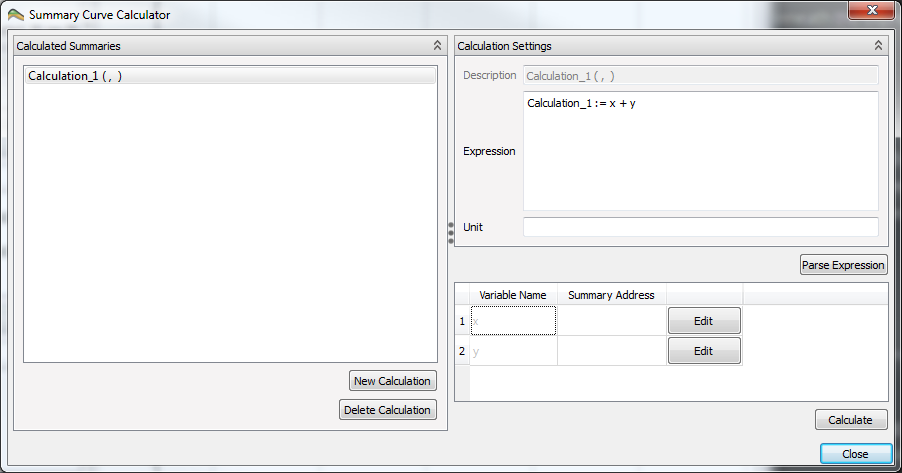# Curve CalculatorThe summary curve calculator is a tool to do relative simple vector calculations on a set of curves. The created curves can be stored for later use in the project.

The calculator can be run by pressing the calculator iconin the menu bar, or by right-clicking on either a summary case or the summary plot collection.

## Calculation Settings

To make a new calculated curve, click on New Calculation. This will add a new calculation to Calculated Summaries. Before choosing which curves to do calculations on, a calculation expression must be made. The default expression Calculation_1 := x + y will do a vector addition on the curves which x and y are placeholders for, and assign it to the calculation Calculation_1. How to assign curves to assign to x and y will be covered in section Summary Address Selection.

### Operators and Functions

Possible operations and functions are found by right-clicking in the expression window. The following tables show all the options available.

#### Assignment Operators

OPERATOR DEFINITION
:= Assign

#### Basic Operators

OPERATOR DEFINITION
- Subtraction
* Multiplication
/ Division
% Modulus
^ Power

#### Scalar Functions

FUNCTION DEFINITION
avg Average
max Maximum
min Minimum
sum Sum

#### Trigonometry Functions

FUNCTION DEFINITION
acosh Inverse hyperbolic cosine (in radians)
asinh Inverse hyperbolic sine (in radians)
atanh Inverse hyperbolic tangent (in radians)
cos Cosine
cosh Hyperbolic cosine
cot Cotangent
csc Cosecant
sec Secant
sin Sine
sinc Sine cardinal
sinh Hyperbolic sine
tan Tangent
tanh Hyperbolic tangent

#### Vector Functions

FUNCTION DEFINITION
abs Absolute value
ceil Rounding up
floor Rounding down
frac Fractional portion of input
log Natural logarithm
log10 Base 10 logarithm
pow Power
round Round x to the nearest integer
sgn Sign of x, -1 where x < 0, +1 where x > 0, else zero
sqrt Square root
trunc Integer portion of input

### Unit

It is possible to add a unit to the calculated curve, in the field Unit beneath the expression field. This will be used as the label on the y-axis when the curve is used in a plot.